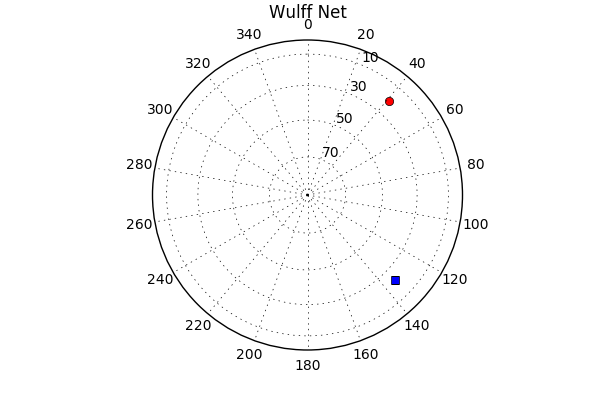In :
import numpy as np
import matplotlib.pyplot as plt
%matplotlib inline
from IPython.display import Image
import pandas as pd

Lecture 22:¶

• Learn some useful tricks about matrix math.

Here's the last figure from Lecture 21:

In :
Image(filename='Figures/wulff.png')
Out:Remember that both of these points are striation directions supposedly found in an ancient bedding plane. Wouldn't it be nice to know the orientation of the plane from these two vectors? Well we can, by using the wonders of matrix math. But first, let's review what planes are in geology and how they are described.

In :
Image(filename='Figures/planes.png')
Out: